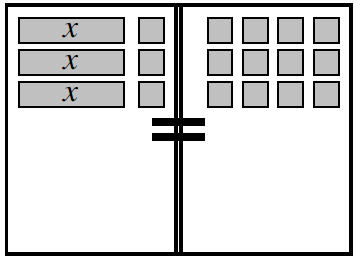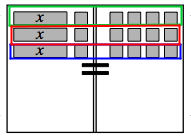### Home > CC2MN > Chapter 7 > Lesson 7.2.1 > Problem7-55

7-55.

When Lakeesha solved the equation $3(x+1)=12$ from problem 7-54, she reasoned this way:

Since $\mathit{3}$ groups of $\mathit{(x+1)}$ equals 3 groups of $\mathit{4}$, then I know that each group of $\mathit{(x+1)}$ must equal $\mathit{4}$.”

1. Do you agree with her reasoning? Explain.

Recall the Equation Mat from problem 7-54.
Each group of $(x+1)$ corresponds to each group of $4$.2. How can the result of Lakeesha’s reasoning be written?

$x+1=4$

3. Verify that your answer from problem 7-54 will make the equation you wrote in part (b) true.

Substitute your answer from problem 7-54 into the equation above and see if the equation is true.